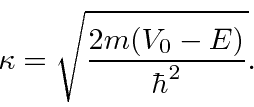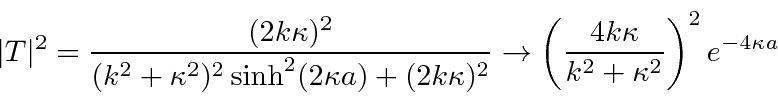### The Potential Barrier

With an analysis of the Potential Barrier problem, we can understand the phenomenon of quantum tunneling.Numbering the three regions from left to right,Again we assume a beam of definite momentum incident from the left and no wave incident from the right. For the solutions outside the barrier,Inside the barrier,This is actually the same as the (unbound) potential well problem with the substitutionin the center region.

The amplitude to be transmitted isWe can compute the probability to be transmitted.An approximate probability is sometimes useful.Classically the transmission probability would be zero. In Quantum Mechanics, the particle is allowed to violate energy conservation for a short timeand so has a chance to tunnel through the barrier.

Tunneling can be applied to cold emission of electrons from a metal, alpha decay of nuclei, semiconductors, and many other problems.

Jim Branson 2013-04-22